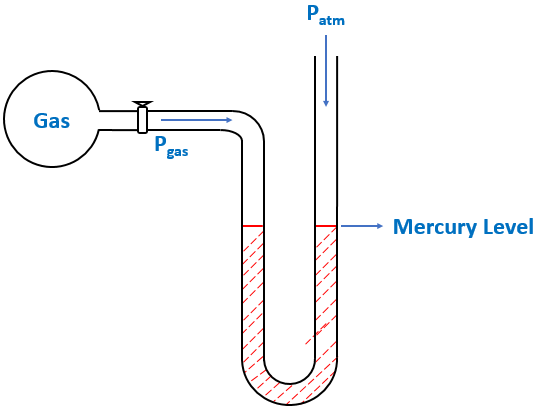# Problem: The barometeric pressure was 749 mmHg when the open end manometer attached to a flask holding a gas read 13.0 mmHg. (mercury level highest on the side connected to the flask.) What is the pressure of the gas in the flask in kilopascals? 1.00 atm = 101.3 kPa

###### FREE Expert Solution
89% (361 ratings)
###### FREE Expert Solution

We’re being asked to determine the pressure of a gas (in kPa) using an open-end manometer.

A manometer allows you to determine the pressure of a gas (Pgas) sample by using the atmospheric pressure (Patm) and the difference in the height of mercury (h).

Different conditions for an open-end manometer are illustrated below:

When Pgas is pushing the same force as Patm$\overline{){{\mathbf{P}}}_{\mathbf{g}\mathbf{a}\mathbf{s}}{\mathbf{=}}{{\mathbf{P}}}_{\mathbf{a}\mathbf{t}\mathbf{m}}}$

When Pgas is pushing harder than Patm

89% (361 ratings)###### Problem Details

The barometeric pressure was 749 mmHg when the open end manometer attached to a flask holding a gas read 13.0 mmHg. (mercury level highest on the side connected to the flask.) What is the pressure of the gas in the flask in kilopascals? 1.00 atm = 101.3 kPa

What scientific concept do you need to know in order to solve this problem?

Our tutors have indicated that to solve this problem you will need to apply the Manometer concept. If you need more Manometer practice, you can also practice Manometer practice problems.

What is the difficulty of this problem?

Our tutors rated the difficulty ofThe barometeric pressure was 749 mmHg when the open end mano...as medium difficulty.

How long does this problem take to solve?

Our expert Chemistry tutor, Dasha took 4 minutes and 18 seconds to solve this problem. You can follow their steps in the video explanation above.

What professor is this problem relevant for?

Based on our data, we think this problem is relevant for Professor Ernst's class at UTAH.